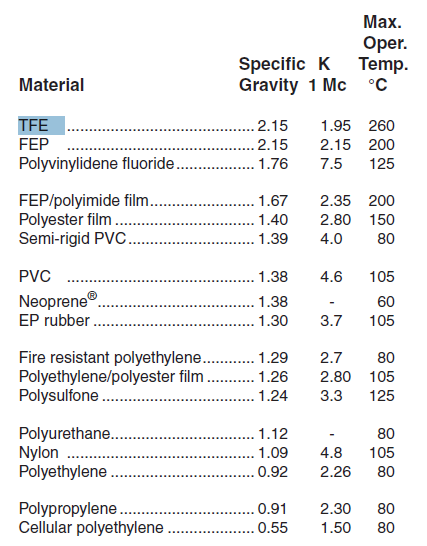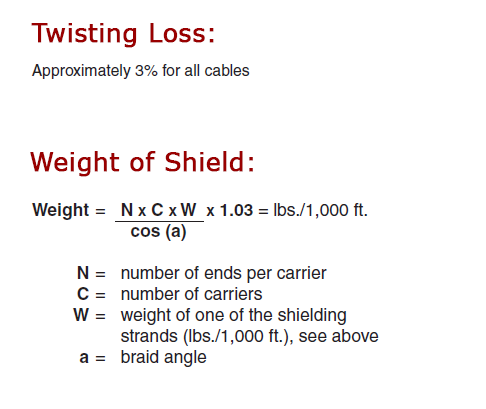Call us now: 01123860572

# Cable Design Formulas

Weight of Conductor:

 Weight = 340.5 D2 GNK = lbs./1,000 ft. D = diameter of conductor in inches G = specific gravity of conductor material; (8.89 for copper, 2.71 for aluminum) N = number of strands K = weight increase factor for stranded conductor. (K = 1 for solid conductor)

 No. of strands K 19 1.02 37 1.026 49 1.03 133 or more 1.04

Weight of Insulation:

 Weight = 340.5 ( D2 - d2 ) G = lbs./1,000 ft. D = diameter over insulation in inches d = diameter over conductor in inches G = specific gravity of insulation

Weight of Jacket:

 Weight = 340.5 ( D2 - d2 ) G = lbs./1,000 ft. D = diameter over jacket in inches d = diameter under jacket in inches G = specific gravity of jacket material

Weight of Tape:

 Weight = 1362 Gt ( ( d+t )+( d+3t ) f ) = lbs./1,000 ft. G = specific gravity of tape t = tape thickness in inches d = diameter of CABLE under tape in inches f = multiplying factor from % lap

 % Lap f 17.5 0.35 25.3 0.5 33.0 0.67 50.0 1.0

Total Weight of CABLED Conductor:

 Weight = N x L x W = lbs./1,000 ft. N = number of conductors L = twisting loss factor = 1.03 W = weight of one conductor

Cabling Factors:

 Number of Conductors Factors Number of Conductors Factors 2 2.0 12 4.155 3 2.154 16 4.7 4 2.414 19 5.0 5 2.7 27 6.155 6 3.0 37 7.0 7 3.0 41 8.0 10 4.0 61 9.0

Use the following formula for other combinations: O.D. = 1.155 x ( Number of Conductors ) x ( Diameter of Individual Conductor )

To determine the approximate O.D. of the finished CABLE, double the wall thickness of the wire, add this figure to the O.D. of the desired stranded conductor and multiply this dimension by the indicated factor for the number of conductors to be in the CABLE. Add 0.025" for a bare, tinned, or silver-plated copper shield of #36 gauge wire; e.g., 6 conductors of 24 gauge, 19/36 stranded, Type E wire with overall shield - 2 x 0.010" (wall) = 0.020" + 0.025" (conductor O.D.) = 0.045" (finished wire). Taking 0.045" (finished wire) x 3 (Factor for 6 conductors) = 0.135". Add the 0.135" to the shield diameter of 0.025" which yields a finished CABLE diameter (no jacket) of 0.160".

Percent of Shield Coverage:
 Percent coverage = ( 2F - F2 ) x 100 F = N P d / Sin (a) N = number of ends (strands) per carrier P = picks per inch D = diameter over dielectric core in inches d = diameter of shielding strand in inches a = the smaller of the two angles between the longitudinal axis of the CABLE and the lay of the braid. C = number of carriers (groups of ends around the diameter of the CABLE in a "two over" "two under" woven basket weave. p = 3.14159265 Tan (a) = 2 p ( D+2d ) P / C

 AWG Size d (inches) W (lbs./1,000 ft) #40 0.0031 0.0291 #38 0.0040 0.0481 #36 0.0050 0.0757 #34 0.0063 0.120 #32 0.0080 0.194 #30 0.0100 0.303

Diameter of Shield:

The formula to determine adders for diameter over the shield diameter of a multi-conductor CABLE is: Shield O.D. = diameter under shield + adder.

 AWG Size (braid) Adder (inches) #40 0.014 #38 0.018 #36 0.022 #34 0.028 #32 0.035 #30 0.044 #28 0.056

Property Values (nominal):